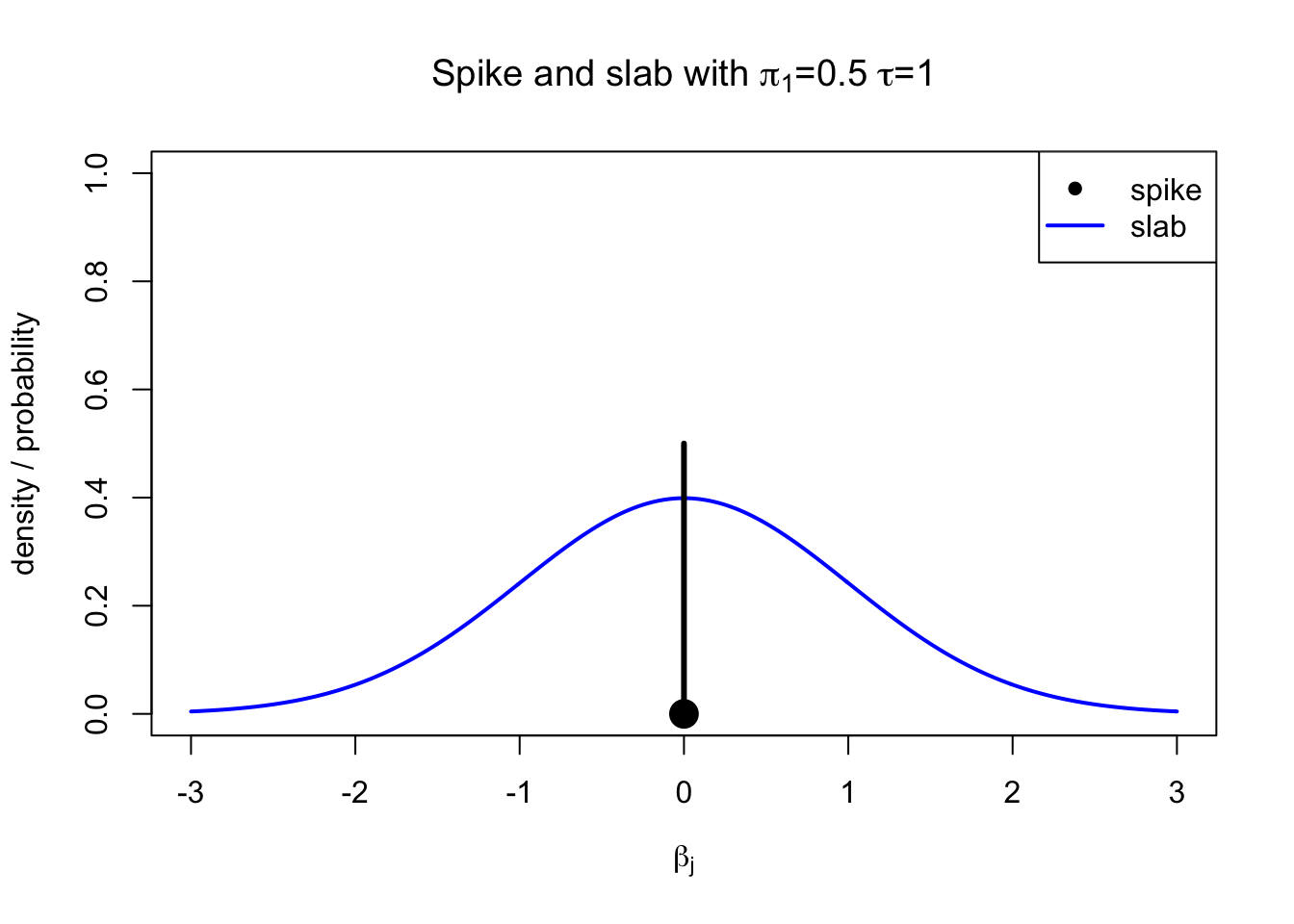In HDS6, we saw that LASSO has a property of setting many of the coefficients to exactly zero at the optimum of the objective function and hence we said that LASSO does variable selection. A technical reason for this behavior was the LASSO’s prior distribution for coefficients that had a sharp peak near zero. In HDS7, we embedded the LASSO model into a fully Bayesian model blasso that allowed us to estimate the full posterior distribution of the coefficients but that was computationally more heavy to apply. Next we will consider conceptually simpler Bayesian models for variable selection, where the prior distribution of a coefficient states that coefficient is non-zero with probability $$\pi_1$$ and has a positive probability mass $$1-\pi_1$$ of being exactly zero.

Spike and slab prior (SSP)

Under SSP, we model individual coefficient $$\beta_j$$ using a mixture distribution $\beta_j\,|\, \pi_1, \tau^2 \sim (1-\pi_1) \delta_0 + \pi_1 \mathcal{N}(0, \tau^2)$ where $$\delta_0$$ is point mass at 0. In other words, $\begin{cases} \beta_j = 0, & \text{with probability } 1-\pi_1,\\ \beta_j \sim \mathcal{N}(0, \tau^2), & \text{with probability } \pi_1. \end{cases}$Suppose we have $$n$$ samples and $$p$$ predictors (columns of $$\pmb X$$) to model outcome vector $$\pmb y$$. Let $$\pmb \gamma = (\gamma_j)_{j=1}^p$$ be vector of binary indicators indicating which variables are non-zero, that is, $\gamma_j = \begin{cases} 1, & \text{if } \beta_j \neq 0,\\ 0, & \text{if } \beta_j = 0. \end{cases}$ We can assign to each predictor, independently, an SSP($$\pi_1,\tau^2$$) prior where the values $$\pi_1$$ and $$\tau^2$$ are shared between the predictors. Value of $$\pi_1$$ could be determined based on which proportion of predictors we expect to be non-zero, and $$\tau^2$$ could be determined based on what magnitude of coefficient values we are expecting. Such model is implemented in BoomSpikeSlab package. (Later we will discuss extensions that estimate $$\pi_1$$ and $$\tau^2$$ from data.)

Let’s try it on the same data we analyzed using Bayesian LASSO in HDS 7 that had $$n=250$$, $$p=30$$ and first 3 variables had an effect size of 0.229 while the remaining 27 were exactly 0. We use prior $$\pi_1 = 5/30 \approx 0.167$$ (by setting expected.model.size = 5) and $$\tau^2=1$$ (by setting prior.beta.sd = rep(1,p)).

set.seed(122)
p = 30
n = 250
phi = 0.05 #variance explained by x_1, should be 0 < phi < 1.
b = rep(c(sqrt(phi / (1-phi)), 0), c(3, p-3)) #effects 1,2,3 are non-zero, see Lect. 0.1 for "phi"
X = scale(matrix(rnorm(n*p), nrow = n)) #cols 1,2,3 of X have effects, other cols are noise
eps = scale(rnorm(n, 0, 1)) #epsilon, error term
y = scale(X%*%b + eps, scale = FALSE) #makes y have mean = 0
library(BoomSpikeSlab)
prior = IndependentSpikeSlabPrior(X, y,
expected.model.size = 5,
prior.beta.sd = rep(1,p))
lm.ss = lm.spike(y ~ X - 1, niter = 1000, prior = prior)
summary(lm.ss)
## coefficients:
##          mean      sd mean.inc sd.inc inc.prob
## X3   3.23e-01 0.06350  0.32300 0.0635    1.000
## X1   2.67e-01 0.07150  0.27100 0.0639    0.985
## X2   2.43e-01 0.07950  0.25100 0.0663    0.966
## X6   9.72e-03 0.03670  0.12000 0.0585    0.081
## X27 -4.60e-03 0.02450 -0.10400 0.0573    0.044
## X23 -3.68e-03 0.02200 -0.08970 0.0648    0.041
## X15 -3.30e-03 0.02220 -0.09180 0.0755    0.036
## X11  2.47e-03 0.01760  0.08230 0.0622    0.030
## X10 -1.26e-03 0.01240 -0.05240 0.0622    0.024
## X14 -7.37e-04 0.01070 -0.03210 0.0645    0.023
## X9  -1.68e-03 0.01570 -0.07620 0.0761    0.022
## X20  9.39e-04 0.01030  0.04470 0.0567    0.021
## X12 -1.31e-03 0.01270 -0.06900 0.0636    0.019
## X5  -8.06e-04 0.01060 -0.04240 0.0663    0.019
## X25  7.40e-04 0.00812  0.04110 0.0460    0.018
## X24 -1.25e-03 0.01220 -0.06930 0.0614    0.018
## X22  2.95e-04 0.00854  0.01640 0.0633    0.018
## X4   6.37e-05 0.00739  0.00354 0.0566    0.018
## X28  1.51e-04 0.01040  0.00887 0.0818    0.017
## X18  8.71e-04 0.01310  0.05120 0.0895    0.017
## X29  5.68e-04 0.00801  0.03550 0.0543    0.016
## X26  1.79e-04 0.00904  0.01190 0.0753    0.015
## X30 -6.47e-04 0.00814 -0.04620 0.0531    0.014
## X21 -1.23e-04 0.00646 -0.00877 0.0559    0.014
## X16  4.56e-04 0.00848  0.03260 0.0664    0.014
## X17 -2.85e-04 0.00856 -0.02190 0.0748    0.013
## X13 -1.47e-04 0.00720 -0.01130 0.0647    0.013
## X19 -4.11e-05 0.00820 -0.00343 0.0781    0.012
## X8  -2.30e-04 0.00472 -0.02300 0.0434    0.010
## X7  -3.41e-04 0.00488 -0.04260 0.0365    0.008
##
## residual.sd =
##    Min. 1st Qu.  Median    Mean 3rd Qu.    Max.
##  0.8700  0.9621  0.9905  0.9937  1.0226  1.1639
##
## r-square    =
##    Min. 1st Qu.  Median    Mean 3rd Qu.    Max.
## -0.0945  0.1551  0.2073  0.2005  0.2520  0.3884

We see that the 3 variables with true effects have posterior inclusion probabilities (PIP) > 0.90 whereas all others have PIPs < 0.10. In the results, we have two sets of posterior means and sds: first from the full posterior distribution and second conditional on variable being included in the model. When the variable has a large PIP, then the two are similar, but for small PIPs, the two differ and the full unconditional mean is a shrunk version of the conditional mean where the shrinkage factor is the PIP. For example, for variable X6 the posterior mean is 0.1200037 conditional on the variable being non-zero, and X6 is non-zero with a posterior probability of 0.081 Thus, the (unconditional) posterior mean is

0.081 $$\cdot$$ 0.1200037 + 0.919 $$\cdot$$ 0 = 0.0097203.

Note that in similar spirit, one could extend the LASSO method to relaxed LASSO where one first runs LASSO to choose the non-zero variables (corresponding to unconditional analysis) and then fits an unpenalized model using only the chosen variables (corresponding to conditional analysis). Statistical inference for such a stepwise procedure is, however, complicated whereas in the Bayesian approach the posterior distribution is directly available and a natural basis for inference.

Let’s visualize the PIPs and posterior distributions of the coefficients.

par(mfrow = c(1,2))
plot(lm.ss) #plots PIPs
plot(lm.ss,"coefficients")#plots coeffs